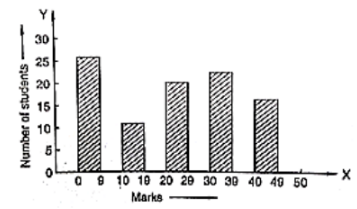# Given below is the bar graph indicating the marks obtained out of 50 in mathematics paper by 100 students.Question:

Given below is the bar graph indicating the marks obtained out of 50 in mathematics paper by 100 students. Read the bar graph and answer the following questions.(i) It is decided to distribute workbooks on mathematics to the students obtaining less than 20 marks, giving one workbook to each of such students. If a workbook costs Rs. 5, what sum is required to buy the workbooks?

(ii) Every student belonging to the highest mark group is entitled to get a prize of Rs. 10. How much amount of money is required for distributing the prize money?

(iii) Every student belonging to the lowest mark-group has to solve 5 problems per day. How many problems, in all, will be solved by the students of this group per day?

(iv) State whether true or false.

(a) 17% students have obtained marks ranging from 40 to 49.

(b) 59 students have obtained marks ranging from 10 to 29.

(v) What is the number of students getting less than 20 marks?

(vi) What is the number of students getting more than 29 marks?

(vii) What is the number of students getting marks between 9 and 40?

(viii) What is the number of students belonging to the highest mark group?

(ix) What is the number of students obtaining more than 19 marks?

Solution:

(i) The number of students obtaining less than 20 marks is 27 + 12 = 39

Hence, the total cost to buy the work books is 5 × 39 = Rs. 195.

(ii) The highest mark group is 40 – 49. The number of students belonging to this group is 17. Hence, the total amount of money required to distribute the prize money is 10 × 17 = Rs. 170

(iii) The lowest mark group is 0-9. The number of students belonging to this group is 27. Hence, the total number of problems will be solved by the students of this group is 5 × 27 = 135

(iv) The total number of students is 100 (given in the question.

(a) The number of students obtaining marks ranging from 40 – 49 is 17.

The percentage of students belonging to this group is (17/100) × 100 = 17 %

Hence, the statement is true.

(b) The number of students obtaining marks ranging from 10 to 29 is 12 + 20 = 32

Hence, the statement is false.

(v) The number of students getting less than 20 marks is 27 + 12 = 39

(vi) The number of students getting more than 29 marks is 24 + 17 = 41

(vii) The number of students getting marks between 9 to 40 is 12 + 20 + 24 = 56

(viii) The number of students belonging to the highest mark group 40 – 49 is 17.

(ix) The number of students obtaining more than 19 marks is 20 + 24 + 17 = 61